×#### Thank you for registering.

One of our academic counsellors will contact you within 1 working day.

Click to Chat

1800-1023-196

+91-120-4616500

CART 0

• 0

MY CART (5)

Use Coupon: CART20 and get 20% off on all online Study Material

ITEM
DETAILS
MRP
DISCOUNT
FINAL PRICE
Total Price: Rs.

There are no items in this cart.
Continue Shopping• Complete Physics Course - Class 11
• OFFERED PRICE: Rs. 2,968
• View Details

Motion in a Straight Line with Acceleration

Table of Content

Uniform Acceleration

Non-Uniform Acceleration

Analysis of Uniformly Accelerated Motion

Velocity Time Graph

Position Time Graph

Velocity Time Graph

Position Time Graph

Acceleration

Acceleration Vector in Non Uniform Motion

Variable Acceleration

Equations of Motion

Refer this Simulation for Motion in a Straight Line

Related Resources

Velocity of a body is defined as the time rate of displacement, where as acceleration is defined as the time rate of change of velocity. Acceleration is a vector quantity. The motion may be uniformly accelerated motion or it may be non-uniformly accelerated, depending on how the velocity changes with time.

Uniform Acceleration

The acceleration of a body is said to be uniform if its velocity changes by equal amounts in equal intervals.

Non-Uniform Acceleration

The acceleration of a body is said to be non-uniform if its velocity changes by unequal amounts in equal intervals of time.

Average velocity

Average acceleration

Illustration:

A particle moves with a velocity v(t) = (1/2)kt2 along a straight line. Find the average speed of the particle in time T.

Solution:

Illustration:

A particle having initial velocity is moving with a constant acceleration 'a' for a time t.

(a)Find the displacement of the particle in the last 1 second.

(b)Evaluate it for u = 2 m/s, a = 1 m/s2 and t = 5 sec.

Solution:

(a)  The displacement of a particle at time t is given s = ut + 1/2at2

At time (t - 1), the displacement of a particle is given by

S' = u (t-1) + 1/2a(t-1)2

So, Displacement in the last 1 second is,

St = S - S'

= ut + 1/2 at2 – [u(t-1)+1/2 a(t-1)2 ]

= ut + 1/2at2 - ut + u - 1/2a(t - 1)2

= 1/2at2 + u - 1/2 a (t+1-2t) =  1/2at2 + u - 1/2at2 - a/2 + at

S = u + a/2(2t - 1)

(b) Putting the values of u = 2 m/s, a = 1 m/s2 and t = 5 sec, we get

S = 2 + 1/2(2 x 5 - 1) = 2 + 1/2 x 9

= 2 + 4.5 = 6.5 m

Illustration:

Position of a particle moving along x-axis is given by x = 3t - 4t2 + t3, where x is in meters and t in seconds.

(a)Find the position of the particle at t = 2 s.

(b)Find the displacement of the particle in the time interval from t = 0 to t = 4 s.

(c)Find the average velocity of the particle in the time interval from t = 2s to t=4s.

(d)Find the velocity of the particle at t = 2 s.

Solution:

(a) x(t) = 3t - 4t2 + t3

=> x(2) = 3 x 2 - 4 x (2)2 + (2)3 = 6 - 4 x 4 + 8 = -2m.

(b) x(o) = 0

X(4) = 3 x 4 - 4 x (4)2 + (4)3 = 12 m.

Displacement = x(4) - x(0) = 12 m.

(c) < v > = X(4)X(2)/(4-2) = (12-(-2))/2 m/s = 7 m/s

(d) dx/dt = 3 - 8t + 3t2

v(2) (dx/dt)2 = 3 - 8 x 2 + 3 x (2)2 = -1m/s

Illustration:

Two trains take 3 sec to pass one another when going in the opposite direction but only 2.5 sec if the speed of the one is increased by 50%. The time one would take to pass the other when going in the same direction at their original speed is

(a) 10 sec                 (b) 12 sec

(c) 15 sec                 (d) 18 sec

Solution:

Using the equation,

t = d/vr

We have,

3 = d/v1+v2

2.5 = d/1.5v1+v2

Solving we get,

v1 = 2d/15 and v2 = d/5

When they are going in same direction,

vr = v2 – v1 = d/15

Thus, t = d/vr = d/(d/15) = 15 s

From the above observation we conclude that, option (c) is correct.

Analysis of Uniformly Accelerated MotionCase-I:
For uniformly accelerated motion with initial velocity u and initial position x0.

Velocity Time Graph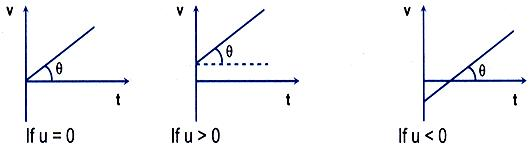In every case tanθ = a0

Position Time Graph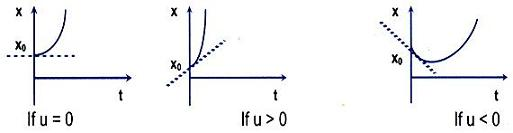Initial position x of the body in every case is x0 (> 0)

Case II:For uniformly retarded motion with initial velocity u and initial position x0.

Velocity Time GraphIn every case tanθ  = -a0

Position Time Graph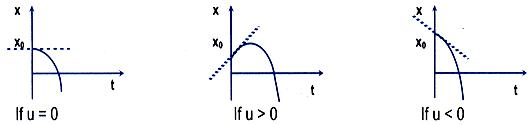Initial position x of the body in every case is x0 (> 0)

Illustration: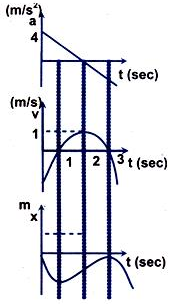A particle is moving rectilinearly with a time varying acceleration a = 4 - 2t, where a is in m/s2 and t is in sec. If the particle is starting its motion with a velocity of -3 m/s from x = 0. Draw a-t, v-t and x-t curve for the particle.

Solution:

a = 4-2t

v = 4t-t2-3

x = 2t2 – t3/3 – 3t

Acceleration

Acceleration is the rate of change of velocity with time. The concept of acceleration is understood in non-uniform motion. It is a vector quantity.

Average acceleration is the change in velocity per unit time over an interval of time.

Instantaneous acceleration is defined as

Acceleration Vector in Non Uniform Motion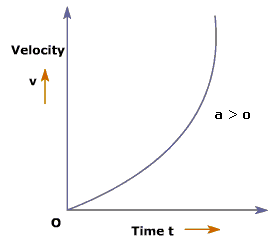Suppose that at the instant t1 a particle as in figure above, has velocity  and at t2, velocity is . The average acceleration   during the motion is defined as

Variable AccelerationThe acceleration at any instant is obtained from the average acceleration by shrinking the time interval closer zero. As Δt tends to zero average acceleration approaching a limiting value, which is the acceleration at that instant called instantaneous acceleration which is vector quantity.

i.e. the instantaneous acceleration is the derivative of velocity.

Hence instantaneous acceleration of a particle at any instant is the rate at which its velocity is changing at that instant. Instantaneous acceleration at any point is the slope of the curve v (t) at that point as shown in figure above.

Equations of Motion

The relationship among different parameter like displacement velocity, acceleration can be derived using the concept of average acceleration and concept of average acceleration and instantaneous acceleration.

When acceleration is constant, a distinction between average acceleration and instantaneous acceleration loses its meaning, so we can write

where   is the velocity at t = 0 and  is the velocity at some time t

Now,

Hence,

…... (2)

This is the first useful equation of motion.

Similarly for displacement

…... (3)

in which  is the position of the particle at t0 and  is the average velocity between t0 and later time t. If at t0 and t the velocity of particle is

…... (4)

From equation (3) and (4), we get,

…... (5)

This is the second important equation of motion.

Now from equation (2), square both side of this equation we get

[Using equation (4)]

Using equation (3), we get,

…... (6)

This is another important equation of motion.

Caution:The equation of motion derived above are possible only in uniformly accelerated motion i.e. the motion in which the acceleration is constant.

Refer this Simulation for Motion in a Straight Line

Illustration:

The nucleus of helium atom (alpha-particle) travels inside a straight hollow tube of length 2.0 meters long which forms part of a particle accelerator. (a) If one assumes uniform acceleration, how long is the particle in the tube if it enters at a speed of 1000 meter/sec and leaves at 9000 meter/sec? (b) What is its acceleration during this interval?

Solution:

(a) We choose x-axis parallel to the tube, its positive direction being that in which the particle is moving and its origin at the tube entrance. We are given x and vx and we seek t. The acceleration ax is not involved. Hence we use equation 3, x = x0 + <v> t.

We get

x = v0 + ½ (vx0) + vx) t, with x0 = 0 or

t = 2x/(vx0+vx),

t = ((2)(2.0 meters))/((1000+9000)meters/sec) = 4.0/10-4 sec    Ans.

(b) The acceleration follows from equation 2, vx = vx0 + axt

=> ax = (v0-vx0)/t = ((9000-1000)meters/sec)/(4.0×10(-4) sec)

= 2.0 × 107 meter/sec2 Ans.

Pause: The above equations of motion are, however, universal and can be derived by using differential calculus as given below:

Or,

Let at t = 0,

then,

Or,

Further we know that,

or

Integrating,

Or,

At, t = 0, x = x0 then c' = x0

Hence,

Thus, we have derived the same equation of motion using calculus.

To understand the use of calculus in solving the kinematics problems we can look into the following illustrations.

Illustration:

The displacement x of a particle moving in one dimension, under the action of a constant force is related to the time t by the equation t = √x + 3 where x is in meter and t is in seconds. Find the displacement of the particle when its velocity is zero.

Solution:

Here t = √x + 3 => √x = t - 3

Squaring both sides, we get x = t2 - 6t + 9,

As we know velocity, v = dx/dt

Hence we get v = dx/dt = 2t - 6

Put v = 0, we get, 2t - 6 = 0

So, t = 3s

When t = 3s, x = t2 - 6t + 9 = 9 - 6(3) + 9 = 0

Hence the displacement of the particle is zero when its velocity is zero.

Illustration:

A particle starts from a point whose initial velocity is v1 and it reaches with final velocity v2, at point B which is at a distance 'd' from point A. The path is straight line. If acceleration is proportional to velocity, find the time taken by particle from A to B.

Solution:

Here acceleration a is proportional to velocity v.

Hence a α v

=> a = kv, where k is constant

=> dv/dt = kv ............... (1)

=> (dv/ds)(ds/dt) = kv => (dv/ds) v = kv

From equation (1),

dv/v = kdt

or,

Or, ln (v2/v1) = kt

Or, t = ln (v2/v1) /k

=  [d ln (v2/v1)/(v2-v1)]The dispalcement remains unaffected due to shifting of origin from one point to the other.

The displacement can have positive, negative or zero value.

The dispalcement is never greater than the actual distance travelled.

The displacement has unit of length.

Velocity can be considered to be a combination of speed and direction.

A change in either speed or direction of motion results in a change in velocity.

It is not possible for a particle to possess zero speed with a non-zero velocity.

A particle which completes one revolution, along a circular path, with uniform speed is said to possesss zero velocity and non-zero speed.

In case a body moves with uniform velocity, along a straight line, its average speed is equal to its instantaneous speed.Question 1:-

If a car at rest accelerates uniformly to a speed of 144 km/h in 20 s, it covers a distance of:

(a) 20 m                 (b) 800 m

(c) 400 m               (d) 1200 m

Question 2:-

If a body of mass 3 kg is dropped downwards, after 1 sec another ball is dropped downwards from the same point. What is the distance between them after 3 sec.

(a) 25 m                (b) 20 m

(c) 50 m                (d) 9.8 m

Question 3:-

Two bodies of different masses ma and mb are dropped from two different heights a and b. The ratio of the times taken by the two to drop through these distances is:

(a) a:b                (b) b:a

(c) a2:b2             (d) √a:√b

Question 4:-

A ball takes t second to fall from a height h1 and 2t seconds to fall from a height h2. Then h1/h2 is:

(a) 0.5               (b) 0.25

(c) 2                  (d) 4Q.1
Q.2
Q.3
Q.4

c

a

d

b

Related Resources

You might like to refer Introduction to Motion in One Dimension.

For getting an idea of the type of questions asked, refer the  Previous Year Question Papers.

To get answer to any question related to motion in a straight line with acceleration click here.

To read more, Buy study materials of Kinematics comprising study notes, revision notes, video lectures, previous year solved questions etc. Also browse for more study materials on Physics here.### Course Features

• 101 Video Lectures
• Revision Notes
• Previous Year Papers
• Mind Map
• Study Planner
• NCERT Solutions
• Discussion Forum
• Test paper with Video Solution